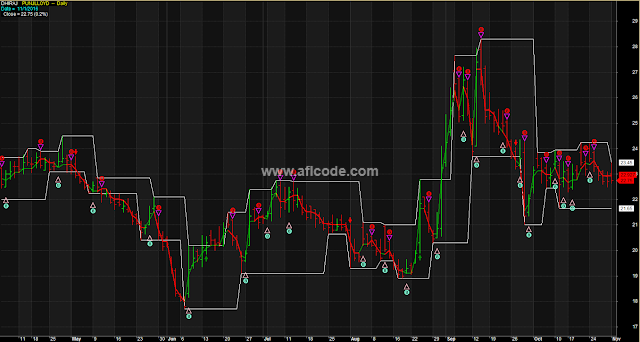### Buy Sell Zone Line ChartBuy Sell Zone Line Chart

```//www.aflcode.com
_SECTION_BEGIN("ORBO 10mt");
priceTitle=EncodeColor(colorYellow)+ StrFormat("    {{NAME}}  --  {{INTERVAL}}" ) + "\n"+ EncodeColor(colorCustom11)+
"Date =  " + Date() ;
ToolTip=StrFormat("  Close = %g (%.1f%%)",C,SelectedValue( ROC( C, 1 ) ));
Title ="DHIRAJ" + priceTitle + "\n" + EncodeColor(colorWhite) + ToolTip;

breakoutime = 100500;
NewDay = Day()!= Ref(Day(), -1);
highestoftheday = HighestSince(newday,H,1);
Lowestoftheday =LowestSince(newday,L,1);
ORBHigh = ValueWhen(afterbreakout0,highestoftheday,1);
ORBLow = ValueWhen(afterbreakout0,lowestoftheday,1);
sellcandidate = Cross(orbhigh,C) AND afterbreakout1 ;

SellCond4=Cross( WMA((L+C+H)/3,9)+0.01,C);
Sell1 = SellCond4 ;
entryprice=WMA((L+C+H)/3,9)+0.01;
ENTRYSELL=WMA((L+C+H)/3,9)-0.01;

Sell = Cross(orblow,C) AND afterbreakout1;

Plot(C,"",colorYellow,styleBar);
PlotShapes( shapeDownArrow * Sell, colorRed,0,H,-12);
//Plot(afterbreakout0,"",colorBlue,styleHistogram|styleOwnScale);

StyleOR=styleNoLine|styleDots+styleThick;

Plot(ORBHigh,"RESISTENCE",colorGreen,StyleOR);
Plot(ORBLow,"SUPPORT",colorRed,StyleOR);

_SECTION_END();

SetBarsRequired(100000,0);
GraphXSpace = 3;

k = Param("K", 0.01, 0, 0.2, 0.001);

Trend = 1;
Revers = C - C*k;
PE = C;

for(i = 1; i < BarCount; i++)
{
if(Trend[i-1] == 1)     //Up Trend
{
if(C[i] > PE[i-1])    // High
{
Trend[i] = 1;
PE[i] = C[i];
Revers[i] = C[i] - C[i]*k;
}
else
{
if(C[i] < Revers[i-1])
{
Trend[i] = -1;
PE[i] = C[i];
Revers[i] = C[i] + C[i]*k;
}
else
{
Trend[i] = 1;
PE[i] = PE[i-1];
Revers[i] = Revers[i-1];
}
}
}
//======================================
else         //Down Trend
{
if(C[i] < PE[i-1])    // Low
{
Trend[i] = -1;
PE[i] = C[i];
Revers[i] = C[i] + C[i]*k;
}
else
{
if(C[i] > Revers[i-1])
{
Trend[i] = 1;
PE[i] = C[i];
Revers[i] = C[i] - C[i]*k;
}
else
{
Trend[i] = -1;
PE[i] = PE[i-1];
Revers[i] = Revers[i-1];
}
}
}
}

Plot(Revers, "NRTR WATR", IIf(Trend == 1, 27, 4), 4);
_SECTION_BEGIN("Price");
SetChartOptions(0,chartShowArrows|chartShowDates);
//_N(Title = StrFormat("{{NAME}} - {{INTERVAL}} {{DATE}}Open %g, Hi %g, Lo %g, Close %g (%.1f%%) Vol " +WriteVal( V, 1.0 ) +" {{VALUES}}", O, H, L, C, SelectedValue( ROC( C, 1 )) ));
//Plot( C, "", ParamColor("Color", colorBlack ), styleNoTitle | ParamStyle("Style") | GetPriceStyle() );
Sell=Cross(Revers,C);
PlotShapes(Sell*shapeHollowDownTriangle,colorCustom12, 0, High, Offset =-10);
PlotShapes(Sell*shapeDigit8,colorRed, 0, High, Offset =25);

_SECTION_END();

// The method of pivot definition is changed.
// Now it does not use zig() or related functions anywhere.

// Stoploss line error, that it moves in both directions after a position is taken,
// is removed. Now stoploss lines are unidirectional.

// To protect profit on long positions, SELL is triggered either when trailing buy stop
// is hit OR when previous lower pivot is cracked. Fresh long position will be initiated
// if uptrend continues after previous high pivot is cracked.

///////////////////////////////////////////////////////////

// This is simple YET powerful pivot based trading system.
// Used only for intraday. Not suitable for EOD data.
// When long, watch crack of close in green line ONLY. Ignore red line.
// When short, watch crack of close in red line ONLY. Ignore green line.
// You will have to tweak the parameters as per your trading style.

//   However, it is very well possible that its performance is not as per actual system
//   suggested by Saint due to programming errors and inadequate knowledge level at my end.
// Warning :  Traders are advised to trade on their own, knowing very well that it is their money.

// User defined parameters.

GraphXSpace = 10; //defines how much extra space should be added above and below graph line (in percent).

dist = 0.25*ATR(10);
Capital=Param("Total capital",100000,10000,1000000,1000);
drawdown=Param("Max. loss per trade as % of Capital", 1.0,0.5,25.0,0.1);
room=Param("Room for S/L as % of Pivot value",0,0,0.02,0.0001);

// Now calculate pivots. Method courtesy : Kenneth (www.traderji.com)

PH= ValueWhen(
(Ref(H,-2) > Ref(H, -4)) AND
(Ref(H,-2) > Ref(H, -3)) AND
(Ref(H,-2) > Ref(H, -1)) AND
(Ref(H,-2) > H), Ref(H,-2));

PL= ValueWhen(
(Ref(L,-2) <= Ref(L, -4)) AND
(Ref(L,-2) <= Ref(L, -3)) AND
(Ref(L,-2) <= Ref(L, -1)) AND
(Ref(L,-2) <= L), Ref(L,-2));

// filter lines

phfilter=PH+(room*PH);
plfilter=PL-(room*PL);

//Uncomment following code if you wish to see the pivot lines.
//Plot(Ref(PH,2), "UpPivot", ParamColor("UpPivot Color",colorRed), styleDashed);
//Plot(Ref(PL,2), "DownPivot",ParamColor("DownPivot Color",colorGreen), styleDashed);

Plot(Ref(Phfilter,2), "Upfilter", ParamColor("upfilter Color",colorWhite), styleLine);
Plot(Ref(Plfilter,2), "Downfilter",ParamColor("dnfilter Color",colorWhite), styleLine);

//Plot(Ref(UpFractal,2), "Up Fractal", ParamColor("Up Fractal Color",colorRed),8);
//Plot(Ref(DownFractal,2), "Down Fractal",ParamColor("Down Fractal Color",colorGreen),8);

/*for (a=4;a<BarCount;a++)
{
if ((H[a-2] >= H[a-4]) AND
(H[a-2] >= H[a-3]) AND
(H[a-2] >= H[a-1]) AND
(H[a-2] >= H[a]))
PlotText("PH", a-2, H[a-2], colorGreen);

if ((L[a-2] <= L[a-4]) AND
(L[a-2] <= L[a-3]) AND
(L[a-2] <= L[a-1]) AND
(L[a-2] <= L[a]))
PlotText("PL", a-2, L[a-2]-dist[a-2], colorRed);
}*/

//Condition for buy : Enter when Close crosses latest pivot high.

//Condition for sell : Exit when previous pivot low is cracked.
Sell = C< (PL-(room*PL));

//Short = Sell;